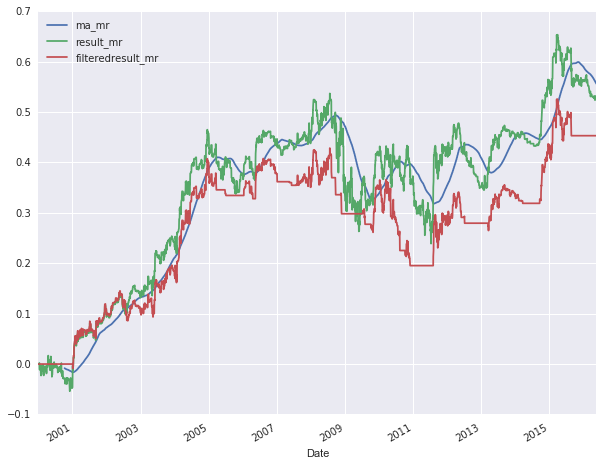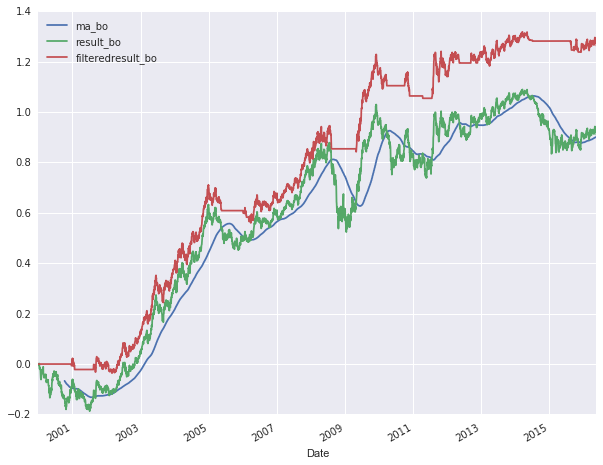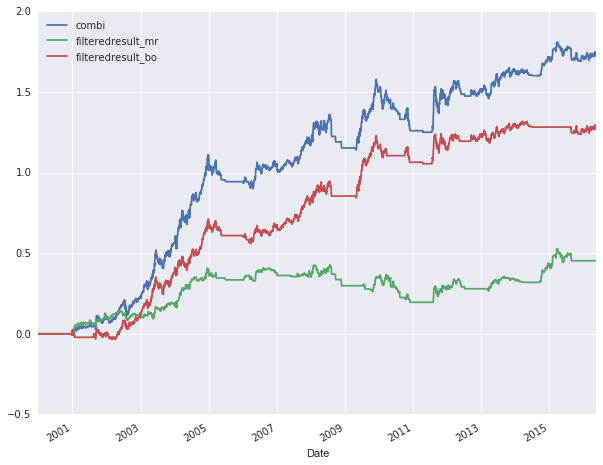### Meanreversion and Breakout research on the EURUSDMean reversion and Breakout strategy combo
Below is a snapshot of some trading research I have been doing.
By no means this is a trade-able strategy as is, but it does show two of the basic trading principles:

• Mean reversion
This is the principle that price tends to return to it's mean after it has moved away from it.
• Breakout
This is the principle that price tends to break away once it has moved more then a certain percentage usually after a period of consolidation.

#### The mean reversion strategy

The strategy that I researched is simple. If the EURUSD moves up by more then 0.5% in one day, then the next day we will go short and close the position by the end of day. And vice versa if the price moves down by more then 0,5%, then we go long and close the position again at the next day's close.

#### The break out strategy

The researched strategy again is very simple. When price moves up by more then 1% in a day, we go long the next day until the close of the next day. And when price moves down by 1% or more, we go short.

#### Filtering times to trade

There are times that certain strategies are more effective then others. There are periods that prices are continuously moving up and down around a mean. And there are other periods that price moves more in a impulsive / corrective mode. To make sure we do not continue trading a strategy while it is no longer performing we apply a filter. The filter I used is a 200 period simple moving average on the daily results of the strategy. So when the strategy is performing below it's average performance, we stop trading that strategy. And we continue trading the strategy as it starts performing above it's mean performance.

#### Vectorized backtest

The backtest below is a so called vectorized backtest done with Python in a Jupyter notebook. This is a quick and convenient way to research trading ideas. I use this way of researching to quickly discard trading ideas. If an idea does not get discarded, I will do an event based back test. If the strategy holds up here, I will paper trade the strategy.

In :
```import pandas as pd
import numpy as np
import seaborn as sns; sns.set()
import datetime
%matplotlib inline
```
In :
```# Read in eurusd daily data from stooq.com
df = pd.read_csv("eurusd_d.csv", index_col="Date", parse_dates=True)
# Select a date range
df = df[(df.index > '2000-1-1') & (df.index <= '2016-12-31')]
```
In :
```# Calculate daily differences
df['diff'] = df['Close'].diff(periods=1)
```
In :
```# Calcultate the cumulative returns
df['cum'] = df['diff'].cumsum()
```
In :
```# Meanreversion
# Setting position long = 1 and short = -1 based on previous day move
delta = 0.005
# If previous day price difference was less than or equal then delta, we go long
# If previous day price difference was more than or equal then delta, we go short
df['position_mr'] = np.where(df['diff'].shift(1) <= -delta,1, np.where(df['diff'].shift(1) >= delta, -1, 0))
df['result_mr'] = (df['diff'] * df['position_mr']).cumsum()
```
In :
```# We will filter execution of our strategy by only executing if our result are above it's 200 day moving average
win =200
df['ma_mr'] = pd.rolling_mean(df['result_mr'], window=win)
filtering_mr = df['result_mr'].shift(1) > df['ma_mr'].shift(1)
df['filteredresult_mr'] = np.where(filtering_mr, df['diff'] * df['position_mr'], 0).cumsum()
# if we do not want to filter we use below line of code
# df['filteredresult_mr'] = (df['diff'] * df['position_mr']).cumsum()
df[['ma_mr','result_mr','filteredresult_mr']].plot(figsize=(10,8))
```
Out:
`<matplotlib.axes._subplots.AxesSubplot at 0x7f4d8ca4c550>`In :
```# Breakout
# Setting position long = 1 and short = -1 based on previous day move
# By setting the delta to negative we are switching the strategy to Breakout
delta = -0.01
# If previous day price difference was less than or equal then delta, we go long
# If previous day price difference was more than or equal then delta, we go short
df['position_bo'] = np.where(df['diff'].shift(1) <= -delta,1, np.where(df['diff'].shift(1) >= delta, -1, 0))
df['result_bo'] = (df['diff'] * df['position_bo']).cumsum()
```
In :
```# We will filter execution of our strategy by only executing if our result are above it's 200 day moving average
win = 200
df['ma_bo'] = pd.rolling_mean(df['result_bo'], window=win)
filtering_bo = df['result_bo'].shift(1) > df['ma_bo'].shift(1)
df['filteredresult_bo'] = np.where(filtering_bo, df['diff'] * df['position_bo'], 0).cumsum()
# df['filteredresult_bo'] = (df['diff'] * df['position_bo']).cumsum()
df[['ma_bo','result_bo','filteredresult_bo']].plot(figsize=(10,8))
```
Out:
`<matplotlib.axes._subplots.AxesSubplot at 0x7f4d8c877358>`In :
```# Here we combine the Meanreversion and the Breakout strategy results
df['combi'] = df['filteredresult_mr'] + df['filteredresult_bo']
df[['combi','filteredresult_mr','filteredresult_bo']].plot(figsize=(10,8))
```
Out:
`<matplotlib.axes._subplots.AxesSubplot at 0x7f4d8c5fb940>`In :
```print("Total return since 2000:",df['combi'][-1] * 100, "%")
```
```Total return since 2000: 171.645 %
```
In :
```def sharpe(serie):
std = serie.std()
mean = serie.mean()
return (mean / std) * 252 ** 0.5
```
In :
```print("Sharpe ratios")
print("Combi", sharpe(df['combi'].diff(periods=1)))
print("Mr", sharpe(df['filteredresult_mr'].diff(periods=1)))
print("Bo", sharpe(df['filteredresult_bo'].diff(periods=1)))
```
```Sharpe ratios
Combi 0.824594193246
Mr 0.41962974772
Bo 0.816470905001
```

This is a different way of researching possible trading strategies in which I can also leverage my coding skills. It is my intention to build up my own algorithmic trading system in which I can continuously research, develop and implement trading strategies and trade these live next to my manually executed trading strategies.